# Solution assignment 02 Addition and subtraction formulas

### Assignment 2

Show: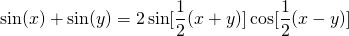### Solution

We know: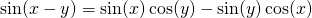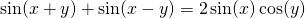Assume: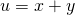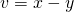Then we expressand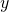in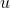and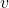, and we get: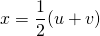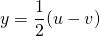Then the formula becomes: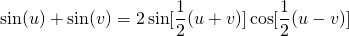When we replacebyandby(what's in a name?), then we get the formula.

0